# How to Calculate Average If Criteria Not Blank/Ignore Blank Cell

In daily work we often need to calculate the average of some numbers based on given conditions or criteria. To calculate average with criteria, we can apply AVERAGEIF of AVERAGEIFS function. AVERAGEIFS function can handle more than one group of criteria range and criteria. In this article, we will show you how to calculate average of numbers whose corresponding cells in another group are not blank. Thus, we need to know some knowledge of AVERAGEIFS function.

In this article, we will let you know the syntax, arguments, and basic usage about AVERAGEIFS function, and apply it to build a formula, also let you know the calculation steps of the formula.

## EXAMPLE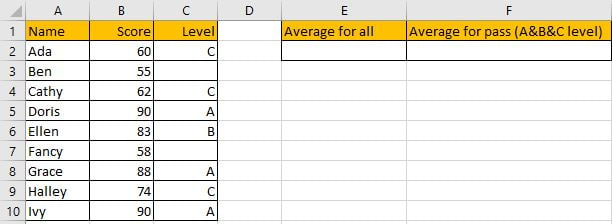Refer to “Score” column, some numbers are listed in range B2:B10. “Level” column is used for providing a level based on scores. In E2 and F2, we calculate the average of all scores and qualified scores separately. To calculate average of all scores, we can directly use AVERAGE function. To calculate average of qualified scores (cell is not blank in Level group), we need to add criteria to filter them, we can apply AVERAGEIFS function, it is also one of the most common used functions in Excel.

First, In C2, enter “=AVERAGE(Score)”. Then press Enter, AVERAGE function returns 73.33333333.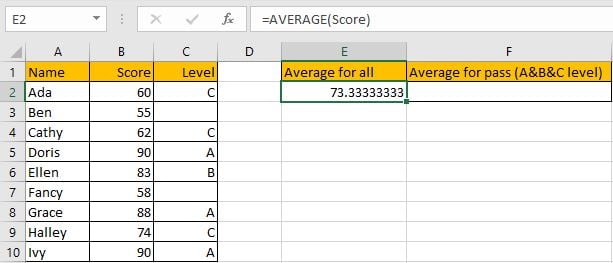You can adjust decimal places by “Increase Decimal” or “Decrease Decimal” in “Number” section.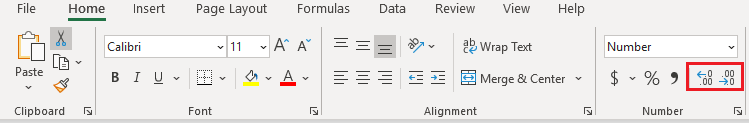Keep clicking on “Decrease Decimal” button till decimal places is ok for you. Keep two decimal places in this case.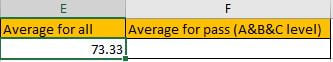Now we can apply AVERGAEIFS function to calculate the average of qualified scores.

Before creating the formula, name range “B2:B10” to “Score”, “C2:C10” to “Level” in Name Box.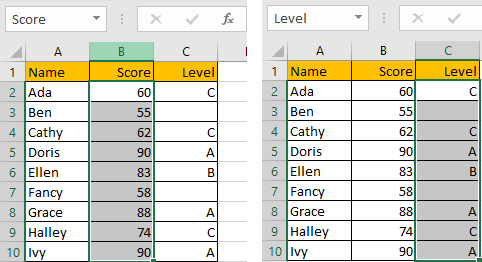## CREATE A FORMULA with AVERAGEIF FUNCTION

### 1. STEPS

Step 1: In F2, enter the formula =AVERAGEIFS(Score,Level,”<>”).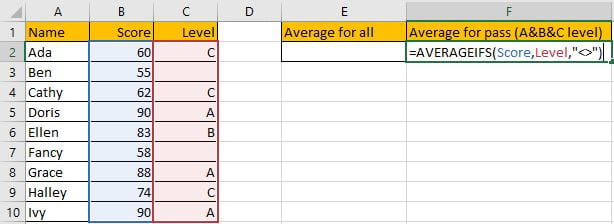Step 2: Press Enter after typing the formula.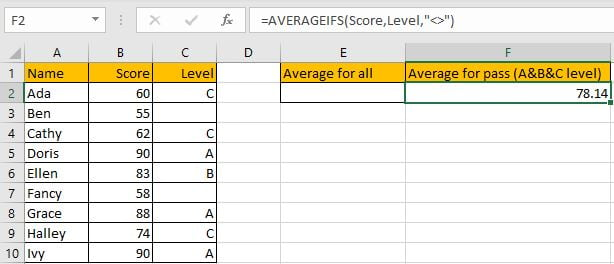Only B2, B4, B5, B6, B8, B9 and B10 meet our given criteria “level is A or B or C”, so we calculate the average of numbers in above cells, total 7 numbers. (60+62+90+83+88+74+90)/7=78.14285714, keep two decimal places 78.14. The formula works correctly.

### 2.FUNCTION INTRODUCTION

AVERAGEIFS function is AVERAGE+IFS. It returns the average of some numbers in a range based on one or more given conditions or criteria.

Syntax:

`=AVERAGEIFS(average_range, criteria_range1, criteria1, [criteria_range2], [criteria2],…)`

It supports wildcards like asterisk ‘*’ and question mark ‘?’, also supports logical expressions like ‘>=’,’<=’. If wildcards or logical operators are required to build criteria, they should be enclosed into double quotes (““). In this case we entered “<>” to represent criteria.

### 3.ALL ARGUMENTS

AVERAGEIFS – Average Range

This example is very simple, we have only one average range A2:A10.

In the formula bar, select named range “Score”, press F9, values in this range are expanded in an array.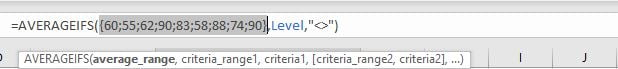AVERAGEIF – Criteria Range

The criteria range is C2:C10.

In the formula bar, select named range “Level”, press F9, values in this range are expanded in an array.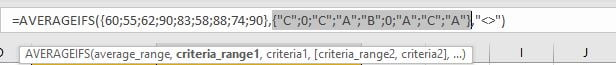AVERAGEIF – Criteria

The criteria in our case is “<>”, in this case it represents cells are not blank. As logical expression is used, so we add “” to quote “<>”.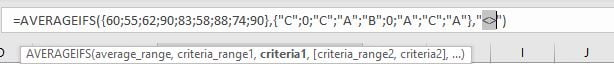### 4.EXPLANATION

After expanding values, the formula is displayed as:

`=AVERAGEIFS({60;55;62;90;83;58;88;74;90},{"C";0;"C";"A";"B";0;"A";"C";"A"},"<>")`

Note: 0 is recorded in this array to represent blank cells.

In the formula, the criteria is “<>” (“not equals to” operator), based on this criteria, cells without any level in “Level” column cannot meet our condition, so for the corresponding values in the average range, they are excluded in calculation.

{60;55;62;90;83;58;88;74;90} -> {60;0;62;90;83;0;88;74;90} – Ignore blank cells

Now this new array only contains numbers. We can calculate the average now (60+62+90+83+88+74+90)/7=78.14.

## SUMMARY

1. AVERAGE function is used for returning the average of some numbers in Excel.

2. AVERAGEIFS function returns the average of some numbers refer to one or more given condition or criteria.

3. AVERAGEIFS can support wildcards, logical expressions.

### Related Functions

• Excel AVERAGE function
The Excel AVERAGE function returns the average of the numbers that you provided.The syntax of the AVERAGE function is as below:=AVERAGE (number1,[number2],…)….
• Excel AVERAGEIF function
The Excel AVERAGEAIF function returns the average of all numbers in a range of cells that meet a given criteria.The syntax of the AVERAGEIF function is as below:= AVERAGEIF (range, criteria, [average_range])….
• Excel AVERAGEIFS function
The Excel AVERAGEAIFS function returns the average of all numbers in a range of cells that meet multiple criteria.The syntax of the AVERAGEIFS function is as below:= AVERAGEIFS (average_range, criteria_range1, criteria1, [criteria_range2, criteria2],…)….

Related Posts

If Cell is This Value or That Value

IF function is frequently used in Excel worksheet to return you expect “true value” or “false value” based on the result of logical test. If you want to see if a cell is A or B, and if one of ...

If Value is Greater Than A Certain Value

IF function is frequently used in Excel worksheet to return you expect “true value” or “false value” based on the logical test result. If you want to see if a value in one cell is greater than a specific value, ...

If Cell is Not Blank

IF function is frequently used in Excel worksheet to return you expect “true value” or “false value” based on the result of created logical test. If you want to see if a cell is blank or not, and leave some ...

If Cell is Blank

IF function is frequently used in Excel worksheet to return you expect “true value” or “false value” based on the result of created logical test. If you want to see if a cell is blank or not, and leave some ...

If Cell Equals Certain Text String

IF function is frequently used in Excel worksheet to return you expect “true value” or “false value” based on the result of created logical test. If you want to see if cell equals a certain text string like “Win”, you ...

If Cell Contains Either Text1 or Text2

IF function is frequently used in Excel worksheet to return “true value” or “false value” based on the logical test result. If you want to see if cell contains certain substring1 like “abc” or substring2 like “def”, and returns true ...

If Cell Contains Certain Text OR Equals Certain Text

IF cell equals certain text IF function is frequently used in Excel worksheet to return “true value” or “false value” based on the logical test result. If you want to test values to see if they equal certain text like ...

VLOOKUP From Another Sheet Not Working

In the previous post, you should know that how to fix or remove the #N/A error when using VLOOKUP formula to lookup value from another sheet. And this post will show you reasons why your VLOOKUP formula is not working ...

If Cell Begins with One of Three Supplied Characters

If you want to test values to see if they begin with some given specific characters like “x”, ”y”, or “z”, you can create a formula with COUNTIF and SUM functions to return results. EXAMPLE You can see “TRUE” or ...

Fix #N/A Error For VLOOKUP From Another Sheet

This post will show you how to fix the #N/A error why it occurs when you extract values from another sheet using VLOOKUP function in Excel 2016,2013,2010 or other Excel versions. How can you correct a #N/A error in VLOOKUP ...

Sidebar